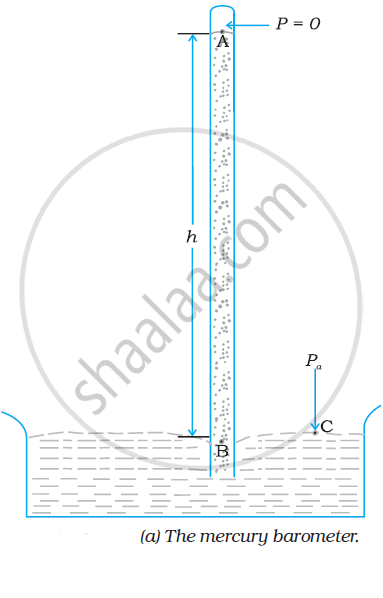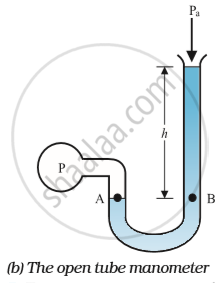# Thrust and Pressure - Atmospheric Pressure and Gauge Pressure

#### notes

Atmospheric Pressure

• The pressure exerted by the weight of the atmosphere.

• The atmosphere is a mixture of different gases. All these gas molecules together constitute some weight. By virtue of this weight, there is some pressure exerted by the atmosphere on all the objects.

• This pressure is known as atmospheric pressure.

• Value of atmospheric pressure at sea level is 1.01 x105

• 1atm = 1.01 x 105Pa

A long glass tube closed at one end and filled with mercury is inverted into a trough of mercury as shown in Fig. This device is known as the ‘mercury barometer’.The space above the mercury column in the tube contains only mercury vapor whose pressure 'P' is so small that it may be neglected. Thus, the pressure at Point A=0.

The pressure inside the column at Point 'B' must be the same as the pressure at Point 'C', which is atmospheric pressure, 'Pa'.

Pa = ρgh

where ρ is the density of mercury and h is the height of the mercury column in the tube.

A pressure equivalent of 1 mm is called a torr (after Torricelli).

1 torr = 133 Pa.

The mm of Hg and torr are used in medicine and physiology.

In meteorology, a common unit is 'bar' and 'millibar'.

1 bar = 105 Pa

Gauge Pressure:

• Pressure difference between the system and the atmosphere.

• From relation P = Pa + ρgh where P= pressure at any point, Pa = atmospheric pressure.

• We can say that Pressure at any point is always greater than the atmospheric pressure by the amount ρgh.

• P - Pa = ρgh where

• P= pressure of the system, Pa= atmospheric pressure,

• (P - Pa) = pressure difference between the system and atmosphere.

• hρg = Gauge pressure.

An open tube manometer is a useful instrument for measuring pressure differences. It consists of a U-tube containing a suitable liquid i.e., a low-density liquid (such as oil) for measuring small pressure differences and a high-density liquid (such as mercury) for large pressure differences.

One end of the tube is open to the atmosphere and the other end is connected to the system whose pressure we want to measure.

The pressure 'P' at 'A' is equal to the pressure at point 'B'. What we normally measure is the gauge pressure, which is (P − Pa), given by equation Pa = ρgh and is proportional to manometer height h.The pressure is the same at the same level on both sides of the U-tube containing a fluid. For liquids, the density varies very little over wide ranges in pressure and temperature and we can treat it safely as a constant for our present purposes. Gases, on the other hand, exhibit large variations of densities with changes in pressure and temperature. Unlike gases, liquids are, therefore, largely treated as incompressible.

Absolute Pressure

• Absolute pressure is defined as the pressure above the zero value of pressure.

• It is the actual pressure that a substance has.

• It is measured against the vacuum.

• Absolute pressure is measured relative to absolute zero pressure.

• It is the sum of atmospheric pressure and gauge pressure.

• P = Pa + hρg where P = pressure at any point, Pa = atmospheric pressure and hρg= gauge pressure.

• Therefore P = Pa + Gauge Pressure. Where P = absolute pressure.

• It is measured with the help of a barometer.

Problem: The density of the atmosphere at sea level is 1.29 kg/m3. Assume that it does not change with altitude. Then how high would the atmosphere extend?

From equation:- "P" = "P"_a +rho"gh"

rho"gh" = 1.29kg/m3 x 9.8m/s2 x hm = 1.01 x 105 Pa

therefore h = 7989m~~8 km

In reality, the density of air decreases with height. So does the value of g. The atmospheric cover extends with a decreasing pressure of over100 km. We should also note that the sea-level atmospheric pressure is not always 760 mm of Hg. A drop in the Hg level by 10 mm or more is a sign of an approaching storm.

Problem:- At a depth of 1000 m in an ocean (a) what is the absolute pressure? (b) What is the gauge pressure? (c) Find the force acting on the window of 20 cm × 20 cm of a submarine at this depth, the interior of which is maintained at sea-level atmospheric pressure. (The density of seawater is 1.03 × 103 kgm-3,g = 10ms–2.)

Here h = 1000m and rho = 1.03 x 10kgm-3

(a) From Eq. P2 - P1 = rhogh, absolute pressure

"P" = "P"_a + rho"gh"

=1.01 xx 10^5"Pa" + 1.03 xx 10^3"kgm"^-3xx10"ms"^-2xx1000"m"

=104.01 xx 10^5"Pa"

~~104"atm"

(b) Gauge pressure is "P" - "P"_a=rho"gh"="P"_g

"P"_g = 1.03 xx 10^3"kg m"^-3xx10"ms"^2xx1000"m"

=103xx10^5  "Pa"

~~103 "atm"

(c) The pressure outside the submarine is P = Pa + ρgh and the pressure inside it is Pa. Hence, the net pressure acting on the window is gauge pressure, Pg = ρgh. Since the area of the window is A = 0.04 m2, the force acting on it is

F = PgA = (103 × 105 Pa) × 0.04 m2 = 4.12 × 105 N

If you would like to contribute notes or other learning material, please submit them using the button below.

### Shaalaa.com

Barometer and Manometer and U - Tube problems [00:54:01]
S
0%